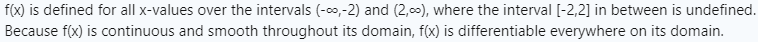# Differentiability

#### Otis

##### Elite Member
Hi Kristina. Part (b) asks where function f is defined, yet you've answered where it's not defined. I would fix that.

For the second question, are you thinking that the function might not be differentiable at some values of x in the domain?

I want to explain further why it is differentiable and not differentiable
Why? The exercise does not ask for that.#### Kristina123

##### New member
Hi Kristina. Part (b) asks where function f is defined, yet you've answered where it's not defined. I would fix that.

For the second question, are you thinking that the function might not be differentiable at some values of x in the domain?

Why? The exercise does not ask for that.I'm thinking that the function f is not differentiable when it approaches closer and closer to -2 and 2 because it seems as if the line is literally a vertical line, but when I graph the derivative of the function it appears that it is differentiable for the all x values of the domain in the function f. So does this mean that the function f doesn't have any areas where it is not differentiable?

#### Otis

##### Elite Member
I'm thinking that the function f is not differentiable when it approaches closer and closer to -2 and 2 because it seems as if the line is literally a vertical line
Thank you for your thoughts, Kristina. As long as x is still approaching -2 from the left or approaching +2 from the right, the function is continuous and differentiable. It doesn't matter how close we are to either asymptote (x=-2 or x=2). The function is not differentiable when x is exactly -2 or 2 (and, as you've indicated) at any value in between.

Therefore, I would cut short your posted answer for the second question, by writing just the first part: f(x) is differentiable everywhere on its domain.

PS: It's not the graph that becomes vertical near -2 and 2. The asymptotes are the only vertical lines. The graph itself is never vertical, even when function values become infinitely large (in absolute value).#### Kristina123

##### New member
Thank you for your thoughts, Kristina. As long as x is still approaching -2 from the left or approaching +2 from the right, the function is continuous and differentiable. It doesn't matter how close we are to either asymptote (x=-2 or x=2). The function is not differentiable when x is exactly -2 or 2 (and, as you've indicated) at any value in between.

Therefore, I would cut short your posted answer for the second question, by writing just the first part: f(x) is differentiable everywhere on its domain.

PS: It's not the graph that becomes vertical near -2 and 2. The asymptotes are the only vertical lines. The graph itself is never vertical, even when function values become infinitely large (in absolute value).Thank you so much for your insightful response. My updated answer for b) is now:•Otis

#### Otis

##### Elite Member
the interval [-2,2] in between is undefined
I understand what you mean, but, technically speaking, the interval [-2,2] is always defined; it's the function that is not defined.•Kristina123

#### Jomo

##### Elite Member
Compute the derivative and figure out its domain

#### Subhotosh Khan

##### Super Moderator
Staff member
I'm thinking that the function f is not differentiable when it approaches closer and closer to -2 and 2 because it seems as if the line is literally a vertical line, but when I graph the derivative of the function it appears that it is differentiable for the all x values of the domain in the function f. So does this mean that the function f doesn't have any areas where it is not differentiable?
Is the function defined when:

|| x|| < 2 .,..?